# SBI PO Prelims Reasoning Question Paper 2019

• Last Updated : 08 Sep, 2022

Directions (1-5): Study the information carefully and answer the questions given below.

Eight persons D, E, F, G, H, I, J, and K were born on two different dates (13th and 22nd) of two different months i.e. February and April of two different years 1992 and 1994. All information is not necessarily in the same order. Only one person was born on one particular date of the given month.

Three persons were born between H and K. Equal number of persons were born after K and before I. H was born on an even number date of a month having more than 28 days.  More than one person was born between I and J who was born on an odd number date. One person was born between G and J. Equal number of persons were born after D and before G. E was not born in the year of D’s birth. Not more than 53 days are there between the birthday of I and H.

Solution(1-5):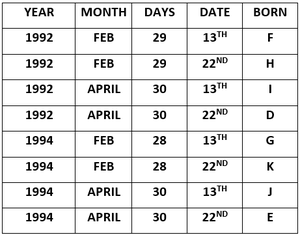Q1. Who among the following person was born on 13th February 1992?

a) D

b) I

c) J

d) F

e) None of these

Solution: d

F was born on 13th February 1992.

Q2. How many persons were born between D and J?

a) Two

b) Three

c) Four

d) Five

e) None of these

Solution: a

Two persons were born between D and J.

Q3. Who among the following person was born on an even number date of the month, which has an even number of days?

a) H

b) K

c) J

d) G

e) All of these

Solution: b

K was born on an even number date of the month, which has an even number of days.

Q4. How many persons were born after E?

a) Two

b) One

c) Four

d) Seven

e) None

Solution: e

None was born after E.

Q5. Which of the following combination is true?

a) 13th February 1992 – I

b) 22nd April 1992- D

c) 13th April 1992- J

d) 22nd April 1992- E

e) None is true

Solution: b

D was born on 22nd April 1992.

Q6. How many such digits are there in the number “6874214593” which will remain at the same position when arranged in ascending order from right to left?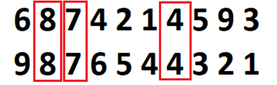a) One

b) Three

c) Two

d) Four

e) Five

Solution: b

Three numerals remain in the same position.

Q7. How many pairs of letters are there in the word ‘SRILANKA’ each of which has as many letters between them in the word as they have between them in the English alphabet?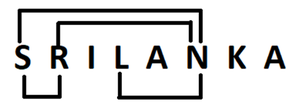(a) one

(b) two

(c) three

(d) four

(e) five

Solution: d

Four pairs of letters are there in the word

Direction (8-10): Point W is 5m west of point Y. Point X is 16m north of point Y. Point W is 25m south of point U. Point U is 10m west of point V. Point V is 9 m north of Point Z.

Solution(8-10):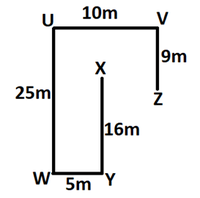Q8. Point Z is at how much distance and in which direction with respect to point X?

(a) 5m, South

(b) 10m, South-east

(c) 5m, East

(d) 10, North-west

(e) 5m, West

Solution: C

Z is 5m East of X.

Q9. What is the direction of Point X with respect to Point U?

(a) north

(b) south-west

(c) south-east

(d) south

(e) north-east

Solution: C

The South-East direction of Point X with respect to Point U.

Q10. What is the direction of Point V with respect to Point W?

(a) north

(b) south-west

(c) north-east

(d) south

(e) north-west

Solution: C

The North-East direction of Point V with respect to Point W.

Directions (11-15) Study the following information carefully and answer the questions given-

Eight persons A, B, C, D, E, F, G and H are sitting in a row. Some of them are facing north and some are facing south. D is second to the right of F. A is second to the left of D.  H sits fifth to the left of C. Only two persons sit between C and F. B sits third to the left of F. B is second to the right of G. C and H did not sit any ends of the row. Both people are sitting at the extreme ends facing opposite directions to each other. Both immediate neighbours of F face in the same direction. E is second to the left of C. A does not face the south direction. H does not face in the north direction. A and D are faced in the same direction.

Solution(11-15):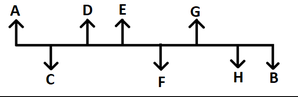Q11. Who among the following sits third to the right of F?

a) B

b) D

c) E

d) A

e) None of these

Solution: e

C sits third to the right of F.

Q12. Which among the following pair sits at the end of the row?

a) D, F

b) E, B

c) A, G

d) A, B

e) None of these

Solution: d

A and B is the pair that sits at the ends of the row.

Q13. How many persons sit to the right of E?

a) Two

b) Three

c) More than three

d) One

e) None of these

Solution: c

Four persons sit to the right of E.

Q14. Who among the following sits fourth to the left of C?

a) E

b) G

c) F

d) B

e) None of these

Solution: b

G sits fourth to the left of C.

Q15. Four of the following are alike in a certain way so form a group, which of the following does not belong to that group?

a) A

b) D

c) E

d) F

e) G

Solution: d

A, D, E, and G are looking in the same direction but F is looking in the opposite direction. So, F does not belong to that group.

Direction (16-18): Study the following information carefully and answer the questions given below:

Six persons M, N, O, P, Q, and R  are arranged according to their weights. Only one person is heavier than M. O is heavier than P but lighter than N. N is not the heaviest. P is not the lightest. M is heavier than Q. Second heaviest person is 65kg and the second lightest person is 45kg.

Solution(16-18)

R > M (65) > N > O> P(45) > Q

Q16. Which of the following person is the lightest?

a) O

b) Q

c) M

d) R

e) None of these

Solution: b

Q is the lightest person.

Q17. What is the possible weight of O?

a) 66kg

b) 43kg

c) 62kg

d) 68kg

e) 38kg

Solution: c

62 kg possible weight of O.

Q18. Which of the following person is the second heaviest?

a) O

b) N

c) M

d) R

e) None of these

Solution :c

M is the second heaviest.

Direction (19-21): In these questions, relationships between different elements are shown in the statements. These statements are followed by two conclusions. Give answer

(a) if only the conclusion I is true

(b) if only conclusion II is true

(c) if either conclusion I or conclusion II is true

(d) if neither conclusion I nor conclusion II is true

(e) if both conclusions I and II are true

Q19. Statement

P > R > F < Y; G > X = M >T> A > L; D > G > Y > E;

Conclusion

I. E > M

II. T < F

Solution: d

Conclusion

I. E > M(False)

II. T < F(False)

if neither conclusion I nor conclusion II is true

Q20. Statement:

X = W < K; H > T = B > K; W > N < S;

Conclusion:

I. T > X

II. N < H

Solution: e

Conclusion:

I. T > X(True)

II. N < H(True)

if both conclusions I and II are true

Q21. Statement:

P > R > L; Z < E = N < A = R, A > B > Y = Q;

Conclusion:

I. L > Y

II. Q < P

Solution: b

Conclusion:

I. L > Y(False)

II. Q < P(True)

if only conclusion II is true

Q22. Four of the following five are alike in a certain way according to the English dictionary form a group, find the one which does not belong to that group?

(a) OLQ

(b) EBG

(c) IFK

(d) URW

(e) WTZ

Solution: e

WTZ does not belong to that group.

Direction (23-26): In each of the questions below are given some statements followed by two conclusions. You have to take the given statements to be true even if they seem to be at variance with commonly known facts. Read all the conclusions and then decide which of the given conclusions logically follows from the given statements disregarding commonly known facts.

(a) If only conclusion I follows.

(b) If only conclusion II follows.

(c) If either conclusion I or II follows.

(d) If neither conclusion I nor II follows.

(e) If both conclusions I and II follow.

Q23. Statements:

All Red are Pink.

Only a few Green is Pink.

All Orange is Green.

Conclusions:

I. Some Orange are not Red.

II. Some Green are Red is a possibility.

(a) If only conclusion I follows.

(b) If only conclusion II follows.

(c) If either conclusion I or II follows.

(d) If neither conclusion I nor II follows.

(e) If both conclusions I and II follow.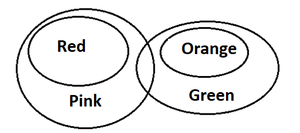Solution: b

Only II Conclusion Follow

Q24. Statements:

Each A are V.

Few V is C.

No A is M.

Conclusions:

I. Some C are M.

II. No M are C.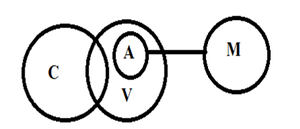(a) If only conclusion I follows.

(b) If only conclusion II follows.

(c) If either conclusion I or II follows.

(d) If neither conclusion I nor II follows.

(e) If both conclusions I and II follow.

Solution: c

If either conclusion I or II follows.

Q25. Statements:

All Paper is White.

No Yellow is White.

Some Blue are Yellow.

Conclusions:

I. All Blue being Paper is a possibility.

II. All Blue can never be White.

(a) If only conclusion I follows.

(b) If only conclusion II follows.

(c) If either conclusion I or II follows.

(d) If neither conclusion I nor II follows.

(e) If both conclusions I and II follow.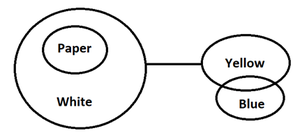Solution: b

Only II Conclusions Follow.

Q26. Statements:

All Fan are AC.

Each Fan is Ball.

No AC is Cooler.

Conclusions:

I. Some Ball can be Cooler.

II. All Cooler being Fan is a possibility.

(a) If only conclusion I follows.

(b) If only conclusion II follows.

(c) If either conclusion I or II follows.

(d) If neither conclusion I nor II follows.

(e) If both conclusions I and II follow.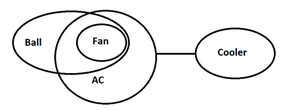Solution: a

Only I conclusion follows.

Directions (27-31): Study the information carefully and answer the question given below.

Nine boxes i.e. M, N, O, P, Q, R, S, T, U are arranged in such a way that one box is placed above the other. The bottom-most box is tagged as 1, the same way the topmost box is tagged as 9.

M is placed 2nd above O. Maximum two boxes are placed between O and N. R is placed 2nd above T.  P is placed 4th above M. M is placed just near to R. Three boxes are placed between R and Q in which Q is placed above R. U is not placed above N.

Solution2(7-31):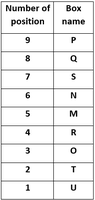Q27. How many boxes are placed between S and O?

a) One

b) Two

c) Three

d) Four

e) None of these

Solution: c

Three boxes are placed between S and O.

Q28. Which of the following box is placed at the Bottom most position?

a) U

b) T

c) Q

d) S

e) None of these

Solution: a

Box U is placed at the bottom-most position.

Q29. Which of the following box is placed one box above box M?

a) R

b) N

c) S

d) Q

e) U

Solution: b

Box N is placed one box above box M.

Q30. Which of the following combinations is correct?

a) Tagged 1- T

b) Tagged 1- S

c) Tagged 1- P

d) Tagged 9- Q

e) None of these

Solution: e

None of the combinations is correct.

Q31. Four of the following five are alike in a certain way and hence form a group. Find the one that does not belong to that group?

a) PQ

b) NM

c) OT

d) SN

e) RM

Solution: e

RM does not belong to that group.

Direction (32-35): Study the following information carefully and answer the following question.

Eight persons S, T, U, V, W, X, Y and Z are sitting around a square table in such a way that those who are sitting at the corners are facing outside and those sitting in the middle are facing inside.

U and T sit opposite each other. Z is not an immediate neighbour of Y. W sits third to the right of Z. One person sits between W and Y. V sits second to the left of U. X sits on one of the corners. Y is not sitting on any side of the square. W is not sitting opposite X. Y is not an immediate neighbour of T.

Solution(32-35):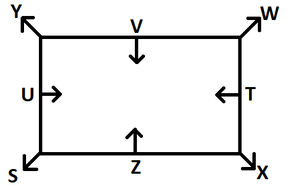Q32. Who sits opposite to V?

a) T

b) X

c) Z

d) S

e) None of these

Solution: c

Z sits opposite V

Q33. Who sits fourth to the right of S?

a) Y

b) U

c) V

d) Z

e) None of these

Solution: e

W sits fourth to the right of S

Q34. How many persons sit between V and S, when counted from the right of S?

a) One

b) Two

c) Three

d) None

e) None of these

Solution: b

Two persons sit between V and S.

Q35. In a way, S is related to X, V is related to T then Y is related to whom?

a. U

b. X

c. Z

d. S

e. T

Solution: d

In the same way, Y is related to S.

My Personal Notes arrow_drop_up